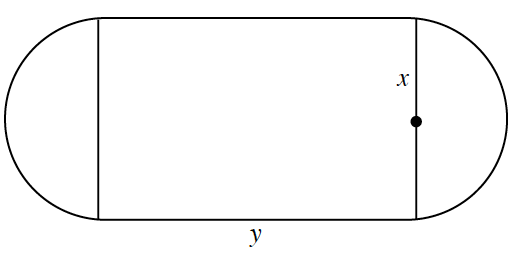### Home > PC3 > Chapter 8 > Lesson 8.1.2 > Problem8-33

8-33.

An indoor physical fitness room consists of a rectangular region with a semicircle on each end. The perimeter of the room is to be a $200$-meter running track. Let $A$ be the area of the rectangular region. Label all parts of the diagram. Note that $x$ is the radius of the circular portion.1. Write an equation for $y$ in terms of $x$.

circumference $=2\pi r$ (or $2\pi x$ in this case)

$200=2y+2\pi x$

2. Express $A$ as a function of $x$.

Use the information from part (a).

$A=xy+\pi x^2$

3. What diameter will create a maximum area for the physical fitness room?

Graph your function from part (b) on a calculator. Locate the point that represents a maximum.